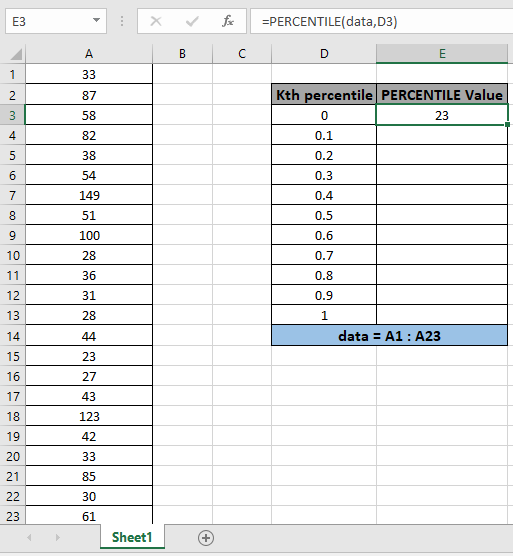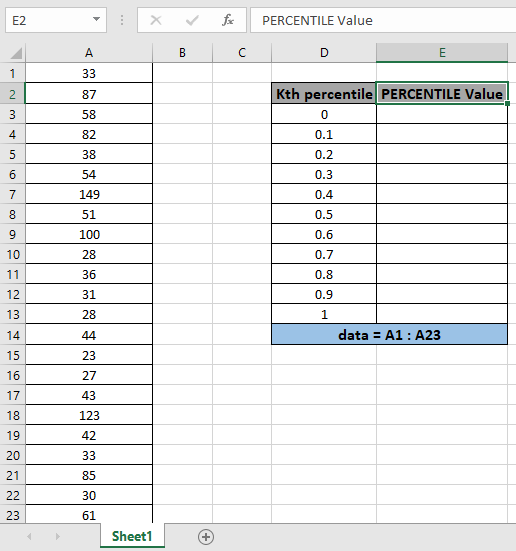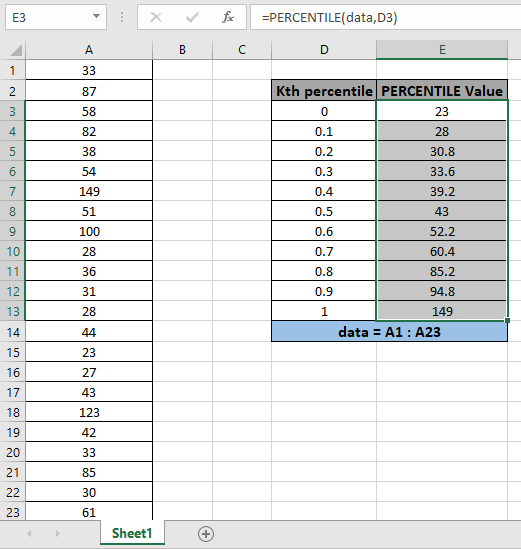# How to use the PERCENTILE function in ExcelIn this article, we will learn How to use the PERCENTILE function in Excel.

What is percentile and percentage ?

Percentage and percentile are two different terms. The key difference between percentage and percentile is the percentage is a mathematical value presented out of 100 and percentile is the percent of values below a specific value. The percentage is a means of comparing quantities. A percentile is used to display position or rank.

For Example. If Isha scored 175 marks out of 200 where highest marks is 180 and lowest marks in 20. Then she is over 90th percentile but has 87.5% percent. Learn to recognize the difference between the two. Let's learn the syntax and example for the PERCENTILE function.

PERCENTILE Function in Excel

PERCENTILE function takes the range of values as array and value of k. And returns the interpolate value from the array.

Syntax:

 =PERCENTILE.EXC (array, k)

array : list of numbers from where to find the percentile of

k : number between 0 & 1 . including 0 & 1 (both)

List of k used in the function

 Value of k Percentage Percentile 0.1 10 % 10th percentile 0.2 20 % 20th percentile 0.3 30 % 30th percentile 0.4 40 % 40th percentile 0.5 50 % 50th percentile 0.6 60 % 60th percentile 0.7 70 % 70th percentile 0.8 80% 80th percentile 0.9 90% 90th percentile

Example :

All of these might be confusing to understand. Let's understand how to use the function using an example. Here we have a set of values as data ( A1 : A23 ) and we need to find some percentile values from the array.Use the formula:

 = PERCENTILE ( data , D3)

data - array given using named range.

D3 - value of k given as cell reference.The function returns the 0th percentile value or minimum value which is 28 .

Now copy the formula to get different percentile values of the range to the remaining cells and formula as shown in the snapshot below.As you can see the results in the snapshot above.

Here are some of the observations gathered using the PERCENTILE function.

Note:

1. The function returns #VALUE! Error if the argument to the function is non numeric.
2. The function returns #NUM! error if the value of k is less than equal to zero or greater than 1.
3. The array argument to the function ignores text, Logic values or Date & Time values.

Hope this article about How to use the PERCENTILE function in Excel is explanatory. Find more articles on calculating values and related Excel formulas here. If you liked our blogs, share it with your friends on Facebook. And also you can follow us on Twitter and Facebook. We would love to hear from you, do let us know how we can improve, complement or innovate our work and make it better for you. Write to us at info@exceltip.com.

Related Articles :

How to use the PERCENTILE.INC function in Excel

How to use the PERCENTILE.EXC function in Excel

How to use the PERCENTRANK function in Excel

How to use the PERCENTRANK.INC function in Excel

How to use the PERCENTRANK.EXC function in Excel

How to use the QUARTILE function in Excel

How to use the QUARTILE.INC function in Excel

How to use the QUARTILE.EXC function in Excel

Popular Articles :

How to use the IF Function in Excel : The IF statement in Excel checks the condition and returns a specific value if the condition is TRUE or returns another specific value if FALSE.

How to use the VLOOKUP Function in Excel : This is one of the most used and popular functions of excel that is used to lookup value from different ranges and sheets.

How to use the SUMIF Function in Excel : This is another dashboard essential function. This helps you sum up values on specific conditions.

How to use the COUNTIF Function in Excel : Count values with conditions using this amazing function. You don't need to filter your data to count specific values. Countif function is essential to prepare your dashboard.

Terms and Conditions of use

The applications/code on this site are distributed as is and without warranties or liability. In no event shall the owner of the copyrights, or the authors of the applications/code be liable for any loss of profit, any problems or any damage resulting from the use or evaluation of the applications/code.